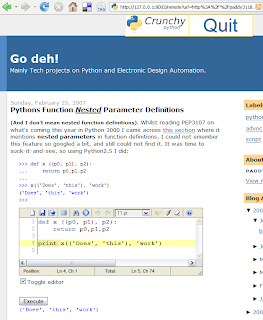# Go deh!

Mainly Tech projects on Python and Electronic Design Automation.

## Saturday, July 21, 2007

### Python makes simple solutions.

The title is a slogan I thought of to counter those who say Python is simple. My slogan emphasises that :
• Python solves problems.
• Those solutions are simple to understand.
• The code produced is simple to maintain.

## Wednesday, July 11, 2007

### I've been Crunched!

There is a new crunchy out. I just downloaded the release, unzipped to a directory, then double-clicked crunchy.py, and Firefox opened a new page of delight. Five minutes of reading and I was able to open this very blog and have all the Python code come alive. On this entry, I was able to re-run the example and have new times printed - in the browser, with just a click of an (added) button!
If I had a new algorithm I could have used the edit window supplied to write and test it as well as save my changes.

The added editor comes pre-loaded with my example code. The new execute button, when clicked inserts the output of running the code from the editor below it. (click on the image for a larger view).Neat!

## Sunday, July 01, 2007

### bit twiddling extras

As a follow up to this
I commented on what I thought would be a better solution. Richard Jones pointed us at how pyglet solved how to find the net power of two and how to check if a number is a power of two using some nifty bit twiddling.

Will McGugan then timed
both his and pyglets code, but didn't implement my suggestion (I didn't give code).

I have added an implementation of my suggestion and timed all three sets.
The timing shows that although my implementation of is_power_of_2 is fastest by a smidgen; My implementation of next_power_of_2 by iterative comparison of a list is half way between Wills and pyglets implementation, with pyglet the winner.

I have not got timings with psyco.

The code
`setup_1 = """from math import log, ceil def is_power_of_2(n):    return log(n, 2) % 1.0 == 0.0 def next_power_of_2(n):    return (2 ** ceil(log(n, 2)))"""setup_2 = """def next_power_of_2(v):    v -= 1    v |= v >> 1    v |= v >> 2    v |= v >> 4    v |= v >> 8    v |= v >> 16    return v + 1 def is_power_of_2(v):    return (v & (v - 1)) == 0"""setup_3 = """list_of_powers = [x**2 for x in range(32)]set_of_powers = set(list_of_powers)def next_power_of_2(v):    for x in list_of_powers:        if x >= v: return xdef is_power_of_2(n):    return n in set_of_powers"""from timeit import Timert1 = Timer("is_power_of_2(128)", setup_1)t2 = Timer("is_power_of_2(128)", setup_2)t3 = Timer("next_power_of_2(125)", setup_1)t4 = Timer("next_power_of_2(125)", setup_2)t5 = Timer("is_power_of_2(128)", setup_3)t6 = Timer("next_power_of_2(125)", setup_3)print "float math is_power_of_2:", t1.timeit()print "bit-twiddling is_power_of_2:", t2.timeit()print "set-lookup is_power_of_2:", t5.timeit()printprint "float math next power of 2:", t3.timeit()print "bit-twiddling next power of 2:", t4.timeit()print "list-comparison next power of 2:", t6.timeit()`

The Timings
`float math is_power_of_2: 2.33588961726bit-twiddling is_power_of_2: 0.573784377623set-lookup is_power_of_2: 0.54499859619float math next power of 2: 3.06279429369bit-twiddling next power of 2: 2.08060354039list-comparison next power of 2: 2.58548279181`